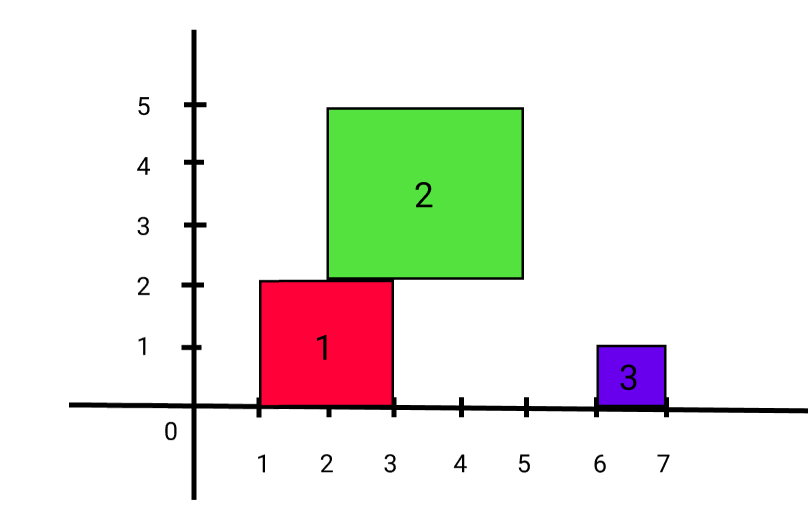New update is available. Click here to update.

# Ninja and Meteorites

Posted: 28 Mar, 2021
Difficulty: Moderate

## PROBLEM STATEMENT

#### For example:

``````Let ‘N’ = 3 and ‘METEORITES’ = [[1, 2], [2, 3], [6, 1]].
The position and size of each meteorite are: </br>
‘Meteorite1’:  At 1 position and size of 2.
‘Meteorite2’:  At 2 position and size of 3.
‘Meteorite3’:  At 6 position and size of 1.
````````````So when the first meteorite falls maximum height till now is 2.
When the second meteorite falls maximum height till now is 5.
When the third meteorite falls maximum height till now is 5.
``````
##### Input Format
``````The first line of input contains an integer ‘T’ which denotes the number of test cases or queries to be run. Then the test cases follow.

The first line of each test case contains an integer ‘N’ representing the number of meteorites.

The next ‘N’ line of each test case contains two single space-separated integers ‘X’ and ‘SIZE’ representing the leftmost X-coordinate and size of the meteorites respectively.
``````
##### Output Format :
``````For each test case, print a single line containing the current highest height of any meteorite after each meteorite falls from space.

The output of each test case will be printed in a separate line.
``````

#### Note:

``````You do not need to print anything; it has already been taken care of. Just implement the given function.
``````
##### Constraints:
``````1 <= ‘T’ <= 10
1 <= ‘N’ <= 1000
1 <= ‘X’ <= 100000
1 <= ‘SIZE’ <= 10000

Where ‘T’ denotes the total number of test cases, ‘N’ represents the number of meteorites,’ X’ denotes the leftmost X-coordinate of the current meteorite and ‘SIZE’ represents the size of the current meteorite.

Time Limit: 1 second
``````